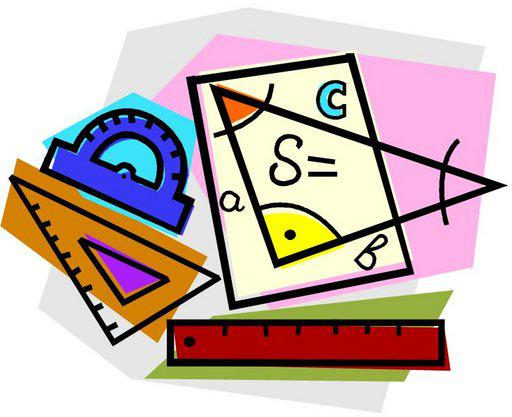COURSE DESCRIPTIONThis course is designed to assist students as they make the transition between the concrete subject of arithmetic and more abstract subjects like algebra and geometry. This is accomplished by working with variables, variable expressions, equations, inequalities, and formulas. Subjects covered in earlier math courses such as fractions, ratios, percents, exponents, roots, and probability are studied in further depth for greater mastery. The students also explore basic algebraic concepts and skills. The course is taught so that a wide range of abilities is challenged through riddles, puzzles, and more complex mathematical problems supplementing the daily coursework. In addition to the specific arithmetic, algebraic and geometric skills and concepts mentioned above, this course aims to develop students’ ability to communicate technical information and mathematical knowledge, which places a heavy emphasis on the processes and reasoning to support answers as well as proper mathematical notation.

CHAPTERSCh.1 – Geometry
Volume and Surface Area of Solids
Cross Sections of Solids
Supplementary and Complementary Angles
Circles: Area and Circumference
Parts of Geometric Figures
Classifying Triangles
Surface Area of Prisms and Cylinders
Circumference of a Circle
Pythagorean Theorem
Using Pythagorean Theorem
Interior Angles of Triangles
Parts of a Circle
Angle Sums in Polygons
Circumference and Area of Circle
Congruent Figures
Calculate Radius and Diameter from Area or Circumference
Identify the Parts of a Solid
Parts of a Circle
Angles and Parallel Lines 7
Using Scale Drawings

Ch.2 – Ratios and Proportions
Recognize and Represent Proportional Relationships
Unit Price Using Proportions
Solving for Percents
Calculating Percents
Ratios & Rates
Estimating Percentages
Proportional Linear Equations

Ch.3 – Number System
Irrational Numbers on a Number Line
Rational Numbers on a Number Line
Operations on Integers
Irrational Numbers on a Number Line
Multiple Representations of Numbers
Integers: Multiplication and Division
Absolute Value
Multiplying and Dividing with Exponents

Ch.4 – Expressions and Equations
Factor Expressions Using the GCF
Estimating Percentages
Writing Algebraic Expressions
Algebraic Patterns
Translating Verbal Expressions
Evaluate Expressions with Rational Numbers
Solving Two-Step Equations with Fractions and Decimals
Areas of Compound Figures
Graphs and Tables
Polynomials

Ch.5 – Exponents: Scientific Notation
Comparing Data by Graph Shape
Compare Summary Statistics
Probability of Dependent or Independent Events
Predicting Outcomes of Experiments
Biased Data
Inequalities
Experimental Probability
Comparing Outcomes to Predictions
Formulate Conclusions Based on Graphs
Finding All Possible Combinations
Range
Predicting Outcomes
Conditional Probability
Comparing Results
Interpreting Graphs
Predicting Results
Sample Space
Tree Diagrams
Experiment Results as Fractions and Ratios
Theoretical Probability

﻿
QQ咨询